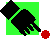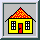Temple University

These lectures are for use in a 2 semester econometrics sequence at the graduate level. Feel free to download any sections you wish, but please give attribution when you do so. Some sections are nearly .5Mb, including the *.gif files which contain equations and diagrams.

Some of the links are straight HTML.  Some links have LiveMath documents embedded in them.  To see these you need to get the viewer from www.livemath.com.  Some links take you to a MathCAD worksheet.  For the MathCAD worksheets you can use the viewer available from www.mathsoft.com.

For a fine collection of econometrics links visit Erasmus University.

Visit the virtual laboratory for probability and statistics.

Topics in the Notes

Click onto expand or collapse the outline

1.A Review of Descriptive Statistics and Probability;
2.Random Variables and Distributions: Discrete and Continuous
3.Notes on Sampling Distributions
4. Estimating Scalar Population Parameters: Methods and Properties
5. Testing Hypotheses about the Mean and Variance
6. An Introduction to Asymptotic Tests: Likelihood Ratio, Wald and Lagrange Multiplier
The Wald, Likelihood Ratio and Lagrange Multiplier Tests stated in terms of the Coefficient of Determination
7.Workouts in Hypothesis Testing
8.A Review of Analysis of Variance and MathCAD examples
9.Simple Regression: Choosing an Estimator, its Properties, Goodness of Fit, and Hypothesis Testing
10.Multiple Regression: More of What You Saw in the Previous Section
11.Generalized Least Squares: The Aitken Estimator, Heteroscedasticity and Autocorrelation
12. Another Pass at Hypothesis Testing in Multiple Regression: The t- and F-statistics; the Wald, Lagrange Multiplier, and Likelihood Ratio Tests; Decision Theoretic Selection Rules
13.Topics in the Pathologies of Multiple Regression
14.Specialized Error Structures
15.Simultaneous Equations Models
16.Time Series
17.   Quantile Regression and a simple MCAD worksheet
18.      Kernel Regression by Prof. Dr. Walter Zucchini
19.    Generalized Method of Moments
20.Appendices

To put to work what you have learned here you might want to visit the Fair Model
Another good site is the Cambridge University Small U.K. Model Distributed Lags

You can find past problem sets and exams HERE.

I'm always happy to hear from people interested in economics and Temple University. You can call me at 215-204-1985. You can send me a FAX at 215-204-8173. Or you can send email to buck@temple.edu